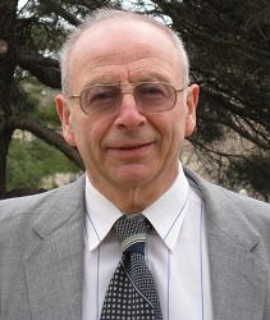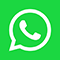Kansas State University, United States

#### Abstract:

The theory of wave scattering by many small impedance particles of arbitrary shapes is developed. The basic assumptions are: a d λ, where a is the characteristic size of particles, d is the smallest distance between the neighboring particles, λ is the wavelength.

This theory allows one to give a recipe for creating materials with a desired refraction coefficient. One can create material with negative refraction: the group veloc- ity in this material is directed opposite to the phase velocity. One can create a material with a desired wave focusing property. Quantum-mechanical scattering by many potentials with small supports is considered.

#### Biography:

Alexander G. Ramm was born in Russia, emigrated to USA in 1979 and is a US citizen. He is Professor of Mathematics with broad interests in analysis, scattering theory, inverse problems, theoretical physics, engineering, signal estimation, tomography, theoretical numerical analysis and applied mathematics.  He is an author of 712 research papers, 20 research monographs and an editor of 3 books. He has lectured in many Universities throughout the world, gave more than 150 invited and plenary talks at various Conferences and had supervised 11 Ph.D students. He was Fulbright Research Professor in Israel and in Ukraine; distinguished visiting  professor in  Mexico and Egypt; Mercator professor in Germany; invited plenary speaker at the 7-th PACOM; he won Khwarizmi international award in 2004 and received many other honors. A.G.Ramm has solved inverse scattering problems with fixed-energy scattering data; with non over-determined scattering data and studied scattering problems with under-determined scattering data. He solved many specific inverse problems and developed new methods in this area. He solved the many-body wave scattering problem when the bodies are small particles of arbitrary shapes and used this theory to give a recipe for creating materials with a desired refraction coefficient. These results attracted attention of the scientists working in nanotechnology. He gave formulas for the scattering amplitude for scalar and electromagnetic waves by small bodies of arbitrary shapes and formulas for the polarizability tensors for such bodies.

He gave a solution to the Pompeiu problem; proved the Schiffer’s conjecture and gave first symmetry results in harmonic analysis. He has developed the Dynamical Systems Method (DSM) for solving linear and non-linear operator equations, especially ill-posed. He developed a random fields estimation theory. A. G. Ramm has developed a theory of convolution equations with hyper-singular integrals. He staudied the spectral properties of the Schroedinger’s operators in domains with non-compact boundaries and gave suffient conditions for the absence of eigenvalues on the continuous spectrum of these operators. A. G. Ramm solved one of the millennium problems concerning the Navier-Stokes problem (NSP) and proved a paradox in the NSP which shows the contradictory nature of the NSP and the non-existence of its solution on the interval t ∈ [0, ∞) for the initial data v0(x) /≡ 0 and f (x, t) = 0.